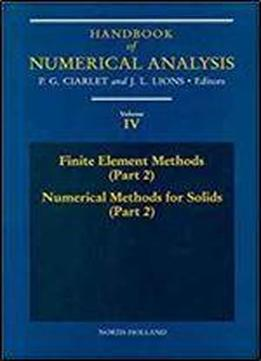# Finite Element Methods (part 2), Numerical Methods For Solids (part 2), Volume 4 (handbook Of Numerical Analysis) by P.G. Ciarlet / 1995 / English / DjVu

This series of volumes aims to cover all the major aspects of numerical analysis, serving as the basic reference work on the subject. Each volume will concentrate on one, two or three particular topics. Each article, written by an expert, is an in-depth survey, reflecting the most recent trends in the field, and is essentially self-contained. The Handbook will cover the basic methods of numerical analysis, under the following general headings: solution of equations in Rn finite difference methods finite element methods techniques of scientific computing and optimization theory and systems science. It will also cover the numerical solution of actual problems of contemporary interest in applied mathematics, under the following headings: numerical methods of fluids numerical methods for solids and specific applications - including meteorology, seismology, petroleum mechanics and celestial mechanics.

views: 289